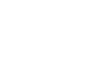How many square feet in an acre? - letsdiskussTrishna Dhanda

Self-Starter!!!!! | Posted | Education

# How many square feet in an acre?

1
0

Student | Posted

Square Feet in an Acre

Acre is defined as the unit of land which is used to measure with the help of length and width. Simultaneously, square feet is commonly used for measuring the property like flats, plot or land areas. The usage of square feet is common in most countries like India, UK, USA, Canada etc. The term square feet is very common in the field of real estate and architecture. There are various flats, plots and land which are measured in square feet and accordingly the cost of the land is estimated. The estimated figure of a square foot in 1 acre is 43560. If anyone is interested in buying the plot in acres, they just have to multiply the square feet by 43560

Dimensions of an Acre

An acre is a measuring unit of a land area. It is also defined in square feet. Acre is not particularly in shape, it can be in any shape starting from rectangle to circle. This is because the area is 43560 square feet

1 acre = 43560 sq ft.

1 acre = 4047 sq metres

1 acre = 4840 sq yards

1 acre = .404686 hectares

The most common shape which is represented as an acre is 1 furlong by 1 chain. Or it can also be stated as 660 feet by 66 feet.Square Feet to Acre

As mentioned all about what an acre and square feet is, further it is important to understand the conversion and how to convert an acre to square feet and vice versa. As we all know, 1 acre is equal to 43560 sq ft., hence to find the cost or to convert multiplication of the acre by the sq feet is done. For instance, if there are 15 acre of land and we have to calculate the square feet area, it is calculated as - 15× 43560= 653400 square feet.

As the real estate business is growing fast nowadays, the people are required to measure the land area or the plot area from what the cost of the area or plot is calculated. As seen above, 1 acre = 43560 sq ft. Likewise, multiplication of any figure with the sq ft will define the estimated cost of that particular area and acre.1
0